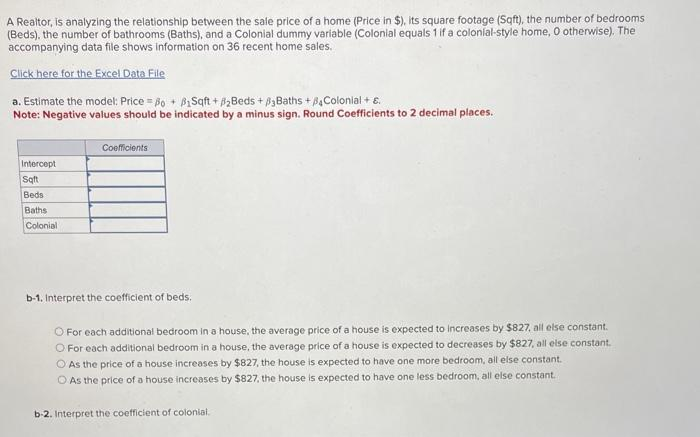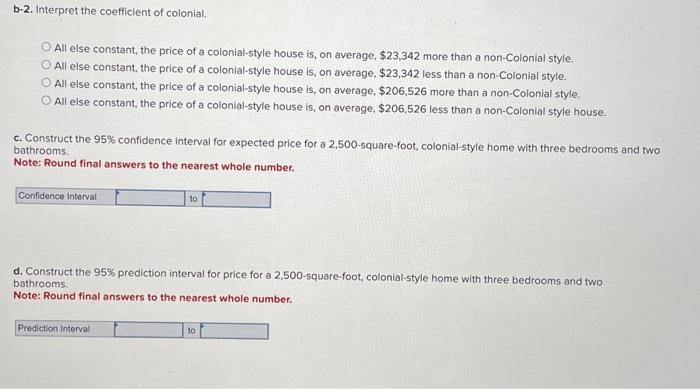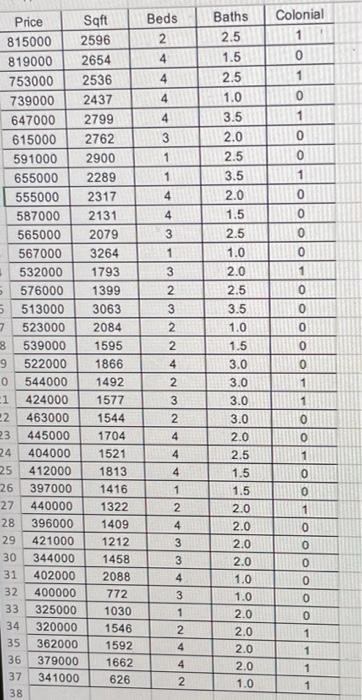Home / Expert Answers / Statistics and Probability / a-realtor-is-analyzing-the-relationship-between-the-sale-price-of-a-home-price-in-its-square-pa741

# (Solved): A Realtor, is analyzing the relationship between the sale price of a home (Price in \$), its square ...A Realtor, is analyzing the relationship between the sale price of a home (Price in \$), its square footage (Sqft), the number of bedrooms (Beds), the number of bathrooms (Baths), and a Colonial dummy variable (Colonial equals 1 if a colonial-style home, 0 otherwise). The accompanying data file shows information on 36 recent home sales. a. Estimate the model: Price $$=\beta_{0}+\beta_{1} \mathrm{Sqft}+\beta_{2}$$ Beds $$+\beta_{3}$$ Baths $$+\beta_{4}$$ Colonial $$+\varepsilon$$ : Note: Negative values should be indicated by a minus sign. Round Coefficients to 2 decimal places. b-1. Interpret the coefficient of beds. For each additional bedroom in a house, the average price of a house is expected to increases by $$\ 827$$, all else constant. For each additional bedroom in a house, the average price of a house is expected to decreases by $$\ 827$$, all eise constant. As the price of a house increases by $$\ 827$$, the house is expected to have one more bedroom, all else constant. As the price of a house increases by $$\ 827$$, the house is expected to have one less bedroom, all else constant. b-2. Interpret the coefficient of coloniai. b-2. Interpret the coefficient of colonial. All else constant, the price of a colonial-style house is, on average, $$\ 23,342$$ more than a non-Colonial style. All else constant, the price of a colonial-style house is, on average, $$\ 23,342$$ less than a non-Colonial style. All else constant, the price of a colonial-style house is, on average, $$\ 206,526$$ more than a non-Colonial style. All else constant, the price of a colonial-style house is, on average, $$\ 206,526$$ less than a non-Colonial style house. c. Construct the $$95 \%$$ confidence interval for expected price for a 2,500-square-foot, colonial-style home with three bedrooms and two bathrooms. Note: Round final answers to the nearest whole number. d. Construct the $$95 \%$$ prediction interval for price for a 2,500-square-foot, colonial-style home with three bedrooms and two bathrooms. Note: Round final answers to the nearest whole number.

We have an Answer from Expert